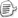Dim Y = Function(f) _
(
Function(h) Function(x) f(h(h))(x)) _
(
Function(h) Function(x) f(h(h))(x))

sig Math.Sin == <double, double>

Dim fact = Y(Function(self) Function(n) If(n = 01, n * self(n - 1)))

sig fact == <int, int>
sig Function(self) Function(n) If (...) == <<int, int>, <int, int>>

sig Y == <YA, YR>
YA == <<a, r>, <a, r>>
YR == <a, r>

sig Y == <<<a, r>, <a, r>>, <a, r>>

= Function(h) Function(x) f(h(h))(x))

sig g(g) == YR == <a, r>

<gA, gR>(<gA, gR>) == <a, r>

gA == <gA, gR> == <gA, <a, r>>
gR == <a, r>

sig g == <<object, <a, r>>, <a, r>>

sig h == <object, <a, r>>
sig x == a

Func<Func<Func<objectobject>, Func<objectobject>>, Func<objectobject>> Y = f =>
{
Func
<Func<object, Func<objectobject>>, Func<objectobject>> g = h => x => f(h(h))(x);

return g(g);
};

static Func<object, Func<T2, T3>> Untype<T1, T2, T3>(Func<T1, Func<T2, T3>> src)
{

return o => o2 => src((T1)o)(o2);
}

Func<Func<Func<objectobject>, Func<objectobject>>, Func<objectobject>> Y = f =>
{
Func
<Func<object, Func<objectobject>>, Func<objectobject>> g = h => x => f(h(h))(x);

return g(Untype(g));
};

Func<Func<objectobject>, Func<objectobject>> my_f = g => n => (int)n == 0 ? 1 : (int)n * (int)g((int)n - 1);

Func
<objectobject> fact = Y(my_f);

int result = (int)fact(8);posted on 2007-11-26 17:14  装配脑袋  阅读(6756)  评论(21编辑  收藏  举报